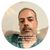Announcements
Applications are open for the 2024 Qlik Luminary Program. Apply by December 15 here.
cancel
Showing results for
Did you mean:Creator II

## 100% Stacked Bar

Hello Every One,

Hope you are doing great. Can someone please help me in finding the logic for 100% stacked bar for below numerator expression.

Dimension Expressions:

1. YEAR (Example Data: 2010,2011,2012,2013,2014). This goes to Category axis.
2. FRUITS (Example Data: Apples, Grapes, Strawberries). This goes to COLOR's

Measure Expression:

Sum({<PLANSIZE={"More than \$50"},CATEGORY={"No"}>}SALES)

Expected Result: I want the sum of all fruits to be 100% for each Year.

I tried the below expression but it is not giving the 100%.

Sum({<PLANSIZE={"More than \$50"},CATEGORY={"No"}>}SALES)/ AGGR(sum(total <YEAR> SALES),YEAR,FRUITS)

Thanks,

-Vidya

1 Solution

Accepted SolutionsMVP

May be -

Sum({<PLANSIZE={"More than \$50"},CATEGORY={"No"}>}SALES)/ AGGR(sum({<PLANSIZE={"More than \$50"},CATEGORY={"No"}>} total <YEAR> SALES),YEAR,FRUITS)

2 RepliesMVP

May be -

Sum({<PLANSIZE={"More than \$50"},CATEGORY={"No"}>}SALES)/ AGGR(sum({<PLANSIZE={"More than \$50"},CATEGORY={"No"}>} total <YEAR> SALES),YEAR,FRUITS)Creator II
Author